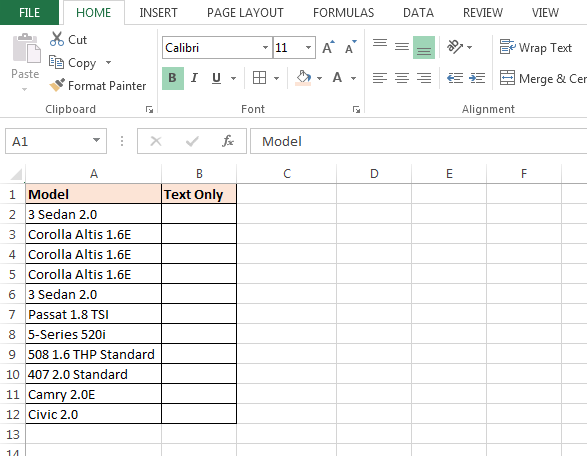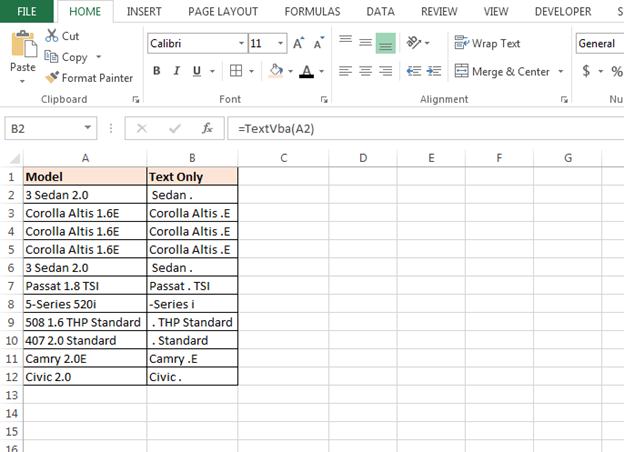# How to Create User Defined Function through VBA

In this article, we are going to learn how to make user defined function to extract the text only from the string through VBA.

User Defined Function: Microsoft Excel allows you to create your own function as per the requirement, we call it User Defined Function. And, we can use User Defined functions like other functions work in Excel.

Let’s understand with a very simple example:

We have a range of strings, from which we only want to retrieve the text value only.To retrieve only text, we will make the VBA code. Through this code we can retrieve the text from any place of the cell. See the below procedure and code you will understand how easy this is:

• Open VBA Page press the key Alt+F11
• Insert a module
• Write the below mentioned code:

```Function TextVba(entry)

For I = 1 To Len(entry)

ThisChar = Mid(entry, I, 1)

Select Case Asc(ThisChar)

Case 48, 49, 50, 51, 52, 53, 54, 55, 56, 57, 58

Case Else

TextVba = TextVba & ThisChar

End Select

Next i

End Function
```

Code Explanation: First, we have given the function name (TextVba). And, then we have given the length of string, then we have used Asc function (this is code Function). Then, we have defined the case. Now, we have defined the condition of TextVba and then Close the Select function.

• Now we will use the function in Excel sheet
• Go to Excel Sheet
• In the cell B2 enter the formula
• =TextVba(A2), press Enter
• Function will return only textIn this way, we can create user defined function and save our time from regular activities.We would love to hear from you, do let us know how we can improve, complement or innovate our work and make it better for you. Write us at info@exceltip.com

1.